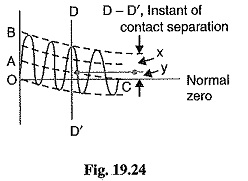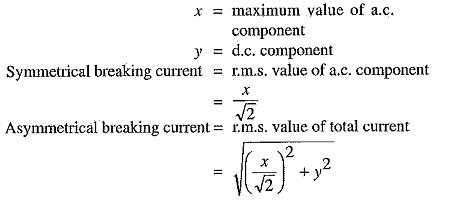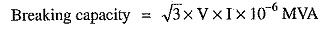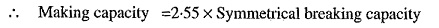## Circuit Breaker Ratings:

A Circuit Breaker Ratings may be called upon to operate under all conditions. However, major duties are imposed on the Circuit Breaker Ratings when there is a fault on the system in which it is connected. Under fault conditions, a circuit breaker is required to perform the following three duties :

• It must be capable of opening the faulty circuit and breaking the fault current.
• It must be capable of being closed on to a fault.
• It must be capable of carrying fault current for a short time while another circuit breaker (in series) is clearing the fault.

Corresponding to the above mentioned duties, the three Circuit Breaker Ratings viz.

1. Breaking Capacity of Circuit Breaker
2. Making Capacity of Circuit Breaker and
3. Short Time Rating.

#### 1. Breaking Capacity of Circuit Breaker:

It is current (r.m.s.) that a circuit breaker is capable of breaking at given recovery voltage and under specified conditions (e.g., power factor rate of rise of restriking voltage).The breaking capacity is always stated at the r.m.s. value of fault current at the instant of contact separation. When a fault occurs, there is considerable asymmetry in the fault current due to the presence of a d.c. component. The d.c. component dies away rapidly, a typical decrement factor being 0.8 per cycle. Referring to Fig. 19.24, the contacts are separated at DD′. At this instant, the fault current hasIt is a common practice to express the breaking capacity in MVA by taking into account the rated breaking current and rated service voltage. Thus, if I is the rated breaking current in amperes and V is the rated service line voltage in volts, then for a 3-phase circuit,In India (or Britain), it is a usual practice to take breaking current equal, to the symmetrical breaking current. However, American practice is to take breaking current equal to asymmetrical breaking current. Thus the American rating given to a Circuit Breaker Ratings is higher than the Indian or British rating.

It seems to be illogical to give breaking capacity in MVA since it is obtained from the product of short-circuit current and rated service voltage. When the short-circuit current is flowing, there is only a small voltage across the breaker contacts, while the service voltage appears across the contacts only after the current has been interrupted. Thus MVA rating is the product of two quantities which do not exist simultaneously in the circuit.

Therefore, the agreed international standard of specifying breaking capacity is defined as the rated symmetrical breaking current at a rated voltage.

#### 2. Making Capacity of Circuit Breaker:

There is always a possibility of closing or making the circuit under short-circuit conditions. The capacity of a breaker to “make” current depends upon its ability to withstand and close successfully against the effects of electromagnetic forces. These forces are proportional to the square of maximum instantaneous current on closing. Therefore, making capacity is stated in terms of a peak value of current instead of r.m.s. value.

The peak value of current (including d.c. component) during the first cycle of current wave after the closure of circuit breaker is known as making capacity.

It may be noted that the definition is concerned with the first cycle of current wave on closing the circuit breaker. This is because the maximum value of fault current possibly occurs in the first cycle only when maximum asymmetry occurs in any phase of the breaker. In other words, the making current is equal to the maximum value of asymmetrical current. To find this value, we must multiply symmetrical breaking current by √2 to convert this from r.m.s. to peak, and then by 1.8 to include the “doubling effect” of maximum asymmetry. The total multiplication factor becomes √2 x 1.8 = 2.55.#### 3. Short Time Rating:

It is the period for which the circuit breaker is able to carry fault current while remaining closed.

Sometimes a fault on the system is of very temporary nature and persists for 1 or 2 seconds after which the fault is automatically cleared. In the interest of continuity of supply, the breaker should not trip in such situations. This means that circuit breakers should be able to carry high current safely for some specified period while remaining closed i.e., they should have proven short-time rating. However, if the fault persists for a duration longer than the specified time limit, the circuit breaker will trip, disconnecting the faulty section.

The short-time rating of a Circuit Breaker Ratings depends upon its ability to withstand (a) the electromagnetic force effects and (b) the temperature rise. The oil circuit breakers have a specified limit of 3 seconds when the ratio of symmetrical breaking current to the rated normal current does not exceed 40. However, if this ratio is more than 40, then the specified limit is 1 second.

Normal current rating: It is the r.m.s. value of current which the circuit breaker is capable of carrying continuously at its rated frequency under specified conditions. The only limitation in this case is the temperature rise of current-carrying parts.

Scroll to Top